# 3D Slice Engine

The initial inspiration for this project came while examining a topographical map that showed contours dividing a landmass into portions of equal altitude. By examining the contour lines defining areas of a similar altitude, we were able to imagine how the landmass would look if we were actually there. These contours gave us insight into the shape and dimensionality of the landmass as perceived from the ground, from the air, or from any other arbitrary point around it. It was from this insight that the Flash 3D slice engine was born.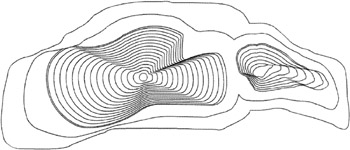In this chapter, we'll go through the development stages of the 3D slice engine, before presenting some examples of the slice engine in action.

## Core Engine Development

Before we get to grips with the construction of the slice engine, it's a good idea to see what the engine can do. As a teaser of what's to come in this chapter, open the files 3D_slice_engine.swf and 3D_perspectives. swf , and take note of the contours and the way in which they react to mouse movement relative to each other. Also note that the combination of contours form the context of an object, in this case a landmass.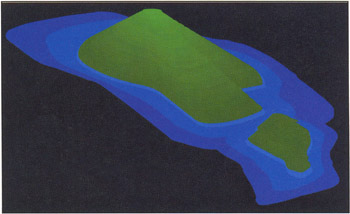### Basic Principles

It's important to understand how the engine works in a general sense before you familiarize yourself with the ActionScript that makes it possible. What we are attempting to do is represent a three-dimensional object in two-dimensional space. The topographic map analogy used earlier is an appropriate starting point, so we'll discuss the process of building a multilayered and interactive landmass.

The fundamental principle is to create a series of layers representing the contours of a landmass object. You then place them relative to one another and use the mouse as a way of rotating the object and defining the angle of the view. An understanding of how the objects and layers are structured will be extremely useful when you get into the scripting side of things.

In order to simulate a 3D object made up of slices, the individual slices have to be skewed. You should be familiar with skewing shapes and symbols using the Free Transform Tool with the Rotate and Skew modifier in the authoring environment. Unfortunately, Flash MX does not have any native support for skewing at runtime (hopefully it will in later versions), so you need to simulate a skewing effect through the nesting of movie clips.

The principle of mimicking runtime skewing in Flash can be a little tricky to understand at first, so it is important that you read and understand the following outline. In this demonstration, we'll be manually replicating the procedure in Flash to help you understand the skewing effect. However, ultimately we'll implement the slice engine functionality through ActionScript to achieve the same result.

1. The shape to be skewed (represented here as a black box) is turned into a movie clip.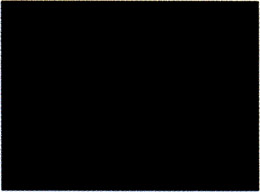2. The movie clip is then placed inside another movie clip (represented by the blue box). This movie clip will be referred to as the container movie clip. The shape we wish to skew is contained or nested within this movie clip.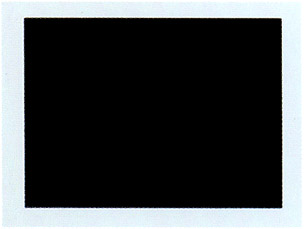3. The nested movie clip (our shape movie clip) is rotated within the container movie clip.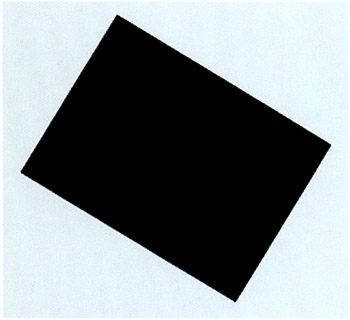4. The container movie clip is then scaled along a single axis (in this case the y-axis).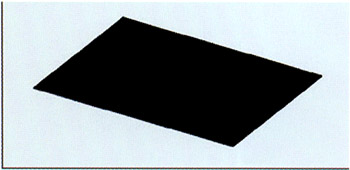5. Next , the container movie clip is rotated in the opposite direction to which we rotated the shape movie clip earlier.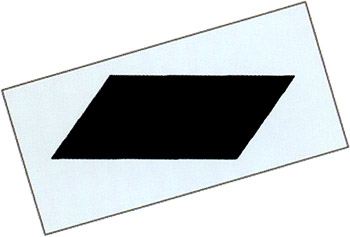6. Finally, the container is scaled the along the y-axis again. If you are trying to manually reproduce this effect, you will have to break apart the container movie clip in order to scale along the y-axis of the stage rather than the movie clip's own y-axis. The diagram shows your final result (left) with the original shape movie clip nested inside your container movie clip (right).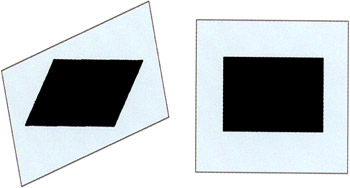It's difficult to see without context, but this simple skewing technique, coupled with some basic trigonometry, forms the core principle behind the slice engine. The engine uses this skewing method in that it builds a stack of movie clips, each containing a nested clip. The nested (or inner) clips are rotated while the clips themselves (the slices) are scaled. By stacking these slices on top of each other and controlling their skewing in relation to each other, we are able to simulate a topographical map or a series of contours and dynamic angles. By placing a number of these slices close enough to each other, we can simulate three-dimensional objects.

Open 3D_perspectives.swf and you'll see a series of contours representing a landmass. The contours have been progressively manipulated to demonstrate change in angle and rotation.### Creating the Object Clip

Now that you've looked at some of the theory behind the Flash 3D slice engine, you're ready to study how it is created. For the first step, you need to create a movie clip with a series of frames containing the contours of your object. As you are producing a landmass, the initial movie clip contours can be created by hand in Flash and do not require any external tools, files, or knowledge.

1. The object clip creation step can be followed by opening the file step1_final.fla , though we recommend that you attempt to create the object from scratch. On the stage, use the Pencil Tool (Y) with the Smooth option selected to create the outline of a landmass approximately 200 pixels wide and 100 pixels high. Fill it with a linear gradient or solid color of your choosing (keeping in mind that as this example is a landmass, you may wish to choose earthy tones).

2. Convert it to a movie clip (F8) and name it slice container . This will be the movie clip that contains your object's layers.3. Next, choose to edit the slice container movie clip by double-clicking on its icon in the Library, and create a keyframe (F6) at frame 15. On this frame, select the shape, reduce it in size , and fill it with a gradient or solid color (make it noticeably different from the color or gradient used in the shape on frame 1).

4. Imagine this shape to be the uppermost point of your landmass, and position and modify it accordingly . Make a shape tween between frame 1 and 15. With the Onion Skin Outlines button switched on, you should be able to recognize the contours of your landmass like so: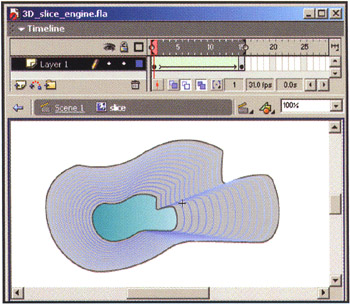### Setting up Assets and Global Variables

In this step, you need to set up the assets and variables required by the slice engine. You need to link your slice container movie clip so that you can use it remotely with ActionScript, and you need to declare some environmental variables that will be used by the slice engine code.

1. Again, this step can be followed by opening the file step2_final.fla . To display the object, the engine needs to attach multiple instances of the slice container movie clip from the Library. To facilitate this, go the Library panel (F11), select slice container , and choose Linkage... from the panel options menu (or right-click/CMD-click for the context-sensitive menu). Check Export for ActionScript ( Export in first frame should also become checked automatically), and then name the identifier inner . Note that this is what you will be referring to the movie clip as when constructing your objects with ActionScript: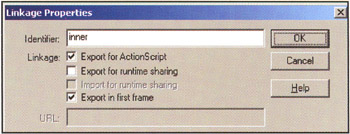2. Next, on frame 1 of your main timeline place the following ActionScript:

`  _quality = "low";   sliceNum = 15;   step = 4;   angle = 20;   scaleFactor = 0.5;   rotSpeed = 0.05;   offsetX = 200;   offsetY = 200;   createEmptyMovieClip("base", 0);   base._x = offsetX;   base._y = offsetY;  `

Before moving on to the next developmental stage, let's break down and analyze the preceding code line by line:

` _quality   =   "low"; `

This engine has the capacity to be rather CPU intensive , so in this first line you turn the movie playback quality to low in order to facilitate a greater number of layers and more responsive interactivity (note that setting _quality to low will stop all antialiasing and bitmap smoothing).

` sliceNum   =   15; `

With this line you define the number of slices that will be used to represent the "landmass."

` step = 4; `

The step is the distance, in pixels, between each slice.

` angle   =   20; `

The angle variable refers to the default angle at which your landmass will be viewed .

` scaleFactor = 0.5; `

The scaleFactor controls the amount that you scale the slices relative to the amount of mouse movement on the y-axis. Depending on the type of object and its environment, this variable could also be perceived as the amount that the object rotates or the elevation of the view of the object (both with respect to the y-axis).

` rotSpeed = 0.05; `

The rotSpeed variable affects the rotational speed of your landmass. In the first instance, you will make the rotation of the landmass reactive to the mouse, so this value will represent rotational speed relative to the distance of the mouse from the center of the landmass. In simple terms, the higher the value of rotSpeed , the faster the rotation.

` offsetX = 200;         offsetY = 200; `

These offsets define the x and y position of the center of the base of the landmass. You then create an empty movie clip named base to attach all of the slices to:

` createEmptyMovieClip  ("base", 0);  `

Finally, you position the base movie clip on the stage. Both offsetX and offsetY are used as the center point from which all your mouse positions will be relative to:

` base._x = offsetX;         base._y = offsetY; `

### Adding Slices to the Base Movie Clip

Now that you've set some global variables, you can go about the creation of the landmass, based upon the slice container movie clip (remember that you gave it the linkage name inner ). Refer to step3_final.fla to follow along with this stage. First, you need to create a loop that will attach multiple instances of inner and will modify their properties. This code will follow on directly from the previous script:

` _quality  =   "low";         sliceNum = 15;         step = 4;         angle = 20;         scaleFactor = 0.5;         rotSpeed = 0.05;         offsetX = 200;         offsetY = 200;         createEmptyMovieClip("base", 0);         base._x = offsetX;         base._y = offsetY;  for (i=0;i<sliceNum;i++) {   base.createEmptyMovieClip("slice"+i,i);   base["slice"+i].attachMovie("inner","inner"+i,i);   base["slice"+i]._y = - i*step*Math.cos(angle*Math.PI/180);   base["slice"+i]._Yscale = Math.sin(angle*Math.PI/180)*100;   base["slice"+i]["inner"+i].gotoAndStop(i+1);   base["slice"+i].myNum = i;   }  `

Here you're using a for loop to build up your slice stack by creating empty movie clips ( " slice" + i ) and attaching the inner clip to them:

` for (i=0;i<sliceNum;i++) {          base.createEmptyMovieClip("slice"+i,i);          base["slice"+i].attachMovie("inner","inner"+i,i); `

As you build the slices on top of each other, you need to space them along the y-axis. As the object is to be built upward from , you need to use a negative value (remember that the y-axis runs from top to bottom in Flash) of the slice number, -i , multiplied by the distance between each slice, step (described in the previous section). Given that step is a distance measured on the y-axis and you are projecting the y-axis from a 3D space to a 2D space, you need to consider the rotation of the object:

` base["slice"+i]._y = - i*step*Math.cos(angle*Math.PI/180); `

Some basic trigonometry is required to do this, and this is best understood if you imagine how the object would appear if viewed along the x-axis: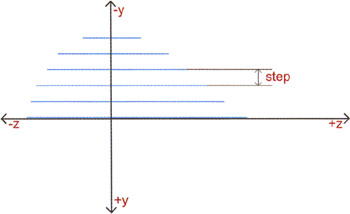As you are measuring each slice from its center, it helps to imagine each slice as the center point of the 3D object. The following diagram indicates the center points of each slice and the separation distance, represented by the variable step :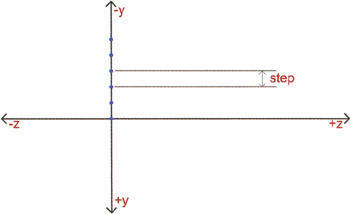As the angle increases when you rotate the object, the projected y aspect of step decreases: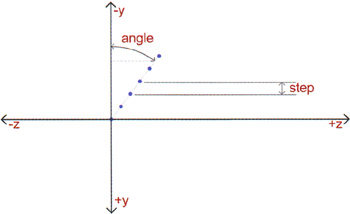The final vertical (y) distance for a certain angle can be calculated using a basic rule of trigonometry with this simple equation:

`  y = step * Cosine (angle*Pi/180)  `

Note that your angle in degrees is multiplied by a factor of Pi/180 to give the angle in radians that Flash requires. Hence, your final line of code, factoring in the y aspect projection of the step variable, looks like this:

` base["slice"+i] ._y = - i*step*Math.cos(angle*Math.PI/180) ; `

The next line of ActionScript follows the same principles as outlined previously:

` base["slice"+i] ._yscale = Math.sin(angle*Math.PI/180)*100; `

In this case, you are calculating the projection of the height of the slice, rather than the projection of step , so you use the sine function instead of cosine.

Now, in order to represent the landmass as a series of layers, you need to tell each instance of inner to go to their respective frames:

` base["slice"+i]["inner"+i].gotoAndStop(i+1); `

You then assign each slice with a variable myNum equal to its order in the slice stack (which is also i ):

` base["slice"+i].myNum = i; `

Although it's not necessary to include this variable in the core engine, it becomes invaluable in later samples as it allows you to identify each slice by an integer value as opposed to a string value (determined by _name ). You can shortcut this step by naming each inner slice i instead of "inner" + i and then referring to _name instead of myNum .

To recap what we've covered so far, at this point your code looks like this:

` quality = "low";         sliceNum = 15;         step = 4;         angle = 20;         scaleFactor = 0.5;         rotSpeed = 0.05;         offsetx = 200;         offsetY = 200;         createEmptyMovieClip("base", 0);         base._x = offsetX;         base._y = offsetY;         for (i = 0;i<sliceNum;i++) {          base.createEmptyMovieClip("slice"+i, i) ;          base["slice"+i].attachMovie("inner","inner"+i, i) ;          base["slice"+i]._y = - i*step*Math.cos (angle*Math.PI/180);          base["slice"+i]._yscale = Math.sin (angle*Math.PI/180)*100;          base["slice"+i]["inner"+i].gotoAndStop(i+1);          base["slice"+i].myNum = i;         } `

Test the movie so far (CTRL/CMD+ENTER), and the end result should look something like this (refer to step3_final.fla if you're having any trouble):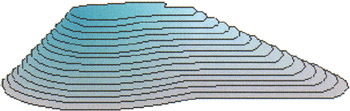You can now start to experiment with the slice engine by changing some of the variables. With the step, angle , and offsetX and offsetY variables, you can control the overall height, viewing angle, and position of the object. For instance, try the following new values:

` sliceNum = 15;  step = 50;   angle = 75;  scaleFactor = 0.5;         rotSpeed = 0.05;  offsetX = 300;   offsetY = 300;  `

These changes result in some specific differences in the look of your slice object: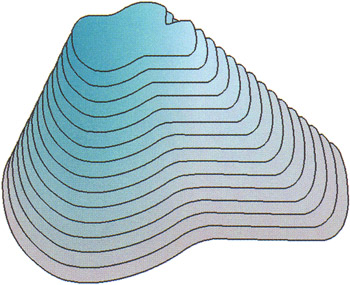You can also experiment with the shape of the object by changing the shapes used on keyframes and the length of the tween used in the slice container movie clip. Make sure that the variable sliceNum always reflects the length of the tweens used in slice container .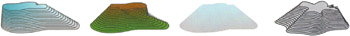There are two basic types of interactive features that you can now add to the functionality of your 3D slice engine: rotational and angular.

#### Rotational Interactivity

Before you add any interactivity, it is important to note that for the sake of an efficient engine you need to create further global variables. In this case, you need to create a function that is triggered by the onEnterFrame event handler on the main timeline.

1. Open the file step4_final.fla and refer to the new ActionScript on the first frame of the main timeline:

`  onEnterFrame = function () {   rot = (this._xmouse-offsetX)*rotSpeed;   };  `

This script is executed every frame and updates variable rot , which is a measure of the x distance between the center of the landmass ( offsetX ) and the current mouse position ( this._xmouse ), multiplied by the rotation speed ( rotSpeed ).

2. After creating variable rot , which is constantly updated, you can add a simple function that defines your object's rotation:

`  function rotateMe() {   this._rotation +=_root.rot;   }  `
3. As discussed earlier, this engine is based on skewing, so in order to effect a skew on each slice, you need to add this function to the onEnterFrame of each instance of inner . You do so within the for loop, as follows:

` for (i=0;i<sliceNum;i++) {     base.createEmptyMovieClip("slice"+i  ,  i);     base["slice"+i).attachMovie("inner","inner"+i,i);     base["slice"+i]._y = - i*step*Math.cos (angle*Math.PI/180);     base["slice"+i]._yscale = Math.sin (angle*Math.PI/180)*100;     base["slice"+i]["inner"+i].gotoAndStop(i+1);  base["slice"+i]["inner"+i].onEnterFrame = rotateMe;  base["slice"+i].myNum = i;    } `
4. If you test the movie with these updates, you'll see that the landmass can now be viewed at the any angle. It rotates around the y-axis in relation to the position of the mouse cursor.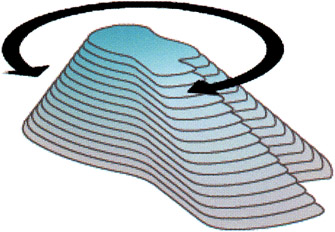### Angular Interactivity

To take things a little further, you can also add angular interactivity.

1. First, you'll add some script that updates your angle value. This works in a similar way to your rotation script but uses the _ymouse coordinates rather than _xmouse . Open the file step5_final.fla and refer to the additional ActionScript on the first frame of the main timeline:

` onEnterFrame = function() {     rot = (this._xmouse - offsetX)*rotSpeed;  angle = (this._ymouse)*scalePactor;  } `
2. Before you add instructions to each instance of slice , note that you no longer require the following lines of code:

` angle = 20;    base["slice"+i]._y = - i*step*Math.cos(angle*Math.PI/180);    base["slice"+i]._yscale = Math.sin(angle*Math.PI/180)*100; `

You're going to dynamically modify the _y position and the _yscale , so these lines are now redundant. Go ahead and delete them (or comment them out).

3. You can now create the function that defines the way in which the dynamic angle is translated into the _yscale of each instance of slice :

`  function scaleMe() {   this._y = - this .myNum*_root.step*Math.cos (_root.angle*Math.PI/180);   this._yscale = Math.sin (_root.angle*Math.PI/180)*100;   }  `

Note that we have created a custom function that will be used by each instance of base ["slice"+i] . We used the this scope to ensure that each instance using this function uses it in reference to its own timeline, thus the term this . The function scaleMe calculates and displays the scale and position of each slice relative to the changing value of variable angle (this relies on the same trigonometry techniques that we explained earlier).

4. Once again, you follow a similar process undertaken when adding rotational interactivity, only in this case you'll be adding an onEnterFrame function for each instance of slice movie clip (remember earlier that you scaled the container and rotated the nested movie clip to simulate skewing):

` for (i=0;i<sliceNum;i++) {     base.createEmptyMovieClip("slice"+i,i);     base["slice"+i].attachMovie("inner","inner"+i,i);     base["slice"+i]["inner"+i].gotoAndStop(i+1);     base["slice"+i]["inner"+i].onEnterFrame = rotateMe;     base["slice"+i].myNum = i;  base["slice"+i].onEnterFrame = scaleMe;  } `
5. On testing this code, you'll see that you've now got an extra degree of angular interactivity.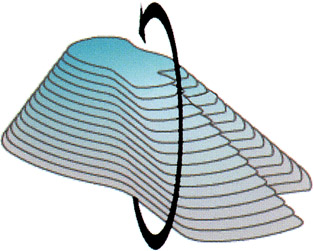That's essentially all that makes up the core components to the slice engine. For completeness, your resulting code should look like this:

` _quality = "low",         sliceNum = 15;         step = 5;         scaleFactor = 0.5;         rotSpeed = 0.05;         offsetX = 200;         offsetY = 200;         createEmptyMovieClip("base", 0);         base._x = offsetX;         base._y = offsetY;         onEnterFrame = function() {          rot = (this._xmouse - offsetX)*rotSpeed;          angle = (this._ymouse) *scaleFactor;         };         for (i=0;i<sliceNum;i++) {          base.createEmptyMovieClip("slice"+i,i) ;          base["slice"+i].attachMovie("inner","inner"+i, i);          base["slice"+i]["inner"+i].gotoAndStop(i+1);          base["slice"+i]["inner"+i].onEnterFrame = rotateMe;          base["slice"+i].myNum = i;          base["slice"+i].onEnterFrame = scaleMe;         }         function rotateMe() {          this.__rotation +=_root.rot;         }         function scaleMe() {          this._y = - this.myNum*_root.step*Math.cos (_root.angle*Math.PI/180);          this._yscale = Math.sin (_root.angle*Math.PI/180) *100;         } `

### Notes and Considerations for using the Slice Engine

As you progressed through the development of the core engine, you might have noticed that there are some limitations. Accordingly, in the following subsections we'll present an outline of these main limitations. This can act as a guide to the "do's and don'ts" when using your Flash 3D slice engine.

#### Processor Intensity

Although the code has been optimized to do the smallest number of calculations possible, there is still the overhead of Flash having to handle multiple objects and their constantly changing position, scale, and rotation. It is a good idea to limit the number of slices to the minimum required to achieve the desired effect. It is also suggested that when using bitmaps, the player quality is turned to low in order to reduce the processor requirements normally used for bitmap smoothing and antialiasing.

In addition, it is important to make sure that there are no unnecessary calculations and that all global variables are calculated on the main timeline rather than every individual instance of slice or inner . The importance of this point will become obvious as you progress through the various implementations of the slice engine in the next section.

In the event that you want to maximize the processing power of the end user machine, it is possible to approximate the processor speed of the machine playing the piece. We have seen many methods of testing processor speed, most of which are based on combining the getTimer() method and some processor-intensive calculations or animation. The time in which it takes the machine to complete a certain process can be used to place it in a range. This classification can then be used to define the number of slices to be used in a given object. If, for example, a machine takes a relatively long time to complete the process, then you could use one-third of the number of slices and use every third frame of the slice container movie clip. If the machine completes the process in ample time, then you know you can use the maximum number of slices and every frame of the movie clip.

#### Angle Limitations

You may also have noted that as the angle of the view approaches , the layers cease rendering. This is because Math. cos (angle*Math. PI/180) = 0 ; therefore, so does the _yscale of each object. It is possible to use angular offsets to render slices at different angles to each other to prevent this, but in most cases, the desired effect is best achieved through a modular 3D box engine as opposed to this slice engine. Feel free to experiment in any case.

#### Z-Order

So far, we have discussed the making of objects so that they can be viewed within an angle variance of 0 to 180 degrees. This has been mostly due to the angle limitations outlined in the previous sections. Taking this into consideration, it is still possible to create an object that can rotate 360 degrees on all axes. To allow this to happen, you need to make sure that your old friend the z-order (or depth ) of your slices is managed appropriately.

When you first built the slice object, you made the uppermost slice have the highest z-order ”that is, it was always on top. You may have experimented with the engine already and discovered that rotating the object less than 0 or greater than 180 degrees gives a strange , reversed mirrored object. To avoid this, you need to reverse the z-order of the slices whenever the angle is less than 0 or greater than 180. To be more accurate, the variable angle is accumulative, and as alternate lots of 180 degrees are passed you need to alternate the z-order.

A simple way of determining alternate lengths of 180 degrees is by dividing the angle by 180, rounding down, and then determining if the result is an odd or even number. For example:

• If the angle is 90 degrees: Divide 90 by 180 to get 0.5, round down to 0, which is even.

• If the angle is 270 degrees: Divide 270 by 180 to get 1.5, round down to 1, and the result is odd. Therefore, you need to swap the z-order.

Now let's look at implementing this into the core engine script. Open the file z-order fix.fla to take a peek at what you are about to achieve. You need to determine the order of the slices every frame, so you'll add some ActionScript to the onEnterFrame function of the root timeline:

` onEnterFrame = function() {          rot = (this._xmouse - offsetX)*rotSpeed;          angle = (this._ymouse) *scaleFactor;  temp = Math.floor(angle/180);   if (Math.floor(temp/2) == temp/2){   order = 1;   }else{   order = -1;   }  }; `

In the first new line, you are using variable temp to divide the angle by 180 and round down to determine a whole integer. You then determine if temp is odd or even by dividing temp by 2 , rounding it down, and then comparing the result to temp divided by 2 (not rounded). All even numbers will return a true result, while all odd numbers will return a false result. From here you can set the variable order , which contains the required order of the slices (either positive or negative).

Now that you know if the order is positive or negative, you can leave it up to each slice to determine its order in the stack. Though there are a number of ways of changing the z-order of the slice stack, we prefer to have each slice determine its own position in the stack. You can do this by adding some script to the scaleMe function, which occurs in onEnterFrame for each slice:

` function scaleMe() {          this._y = - this.myNum*_root.step*Math.cos (_root.angle*Math.PI/180);          this._yscale = Math.sin (_root.angle*Math.PI/180 ) *100;  this.swapDepths(this.myNum*_root.order)  } `

Here you are using each slice's unique identifier myNum and multiplying it by _root. order . In the case of the core engine outline thus far, the resulting z-order is 1 through to 15 if the order is positive, or -15 to -1 if the order is negative. Don't worry about the fact that you are using negative depth values, because Flash will make them positive values while retaining the order of each slice relative to one another.

Test z-order_fix.fla to see the effect of your z-order fix.Flash 3D Cheats Most Wanted
ISBN: 1590592212
EAN: N/A
Year: 2002
Pages: 97